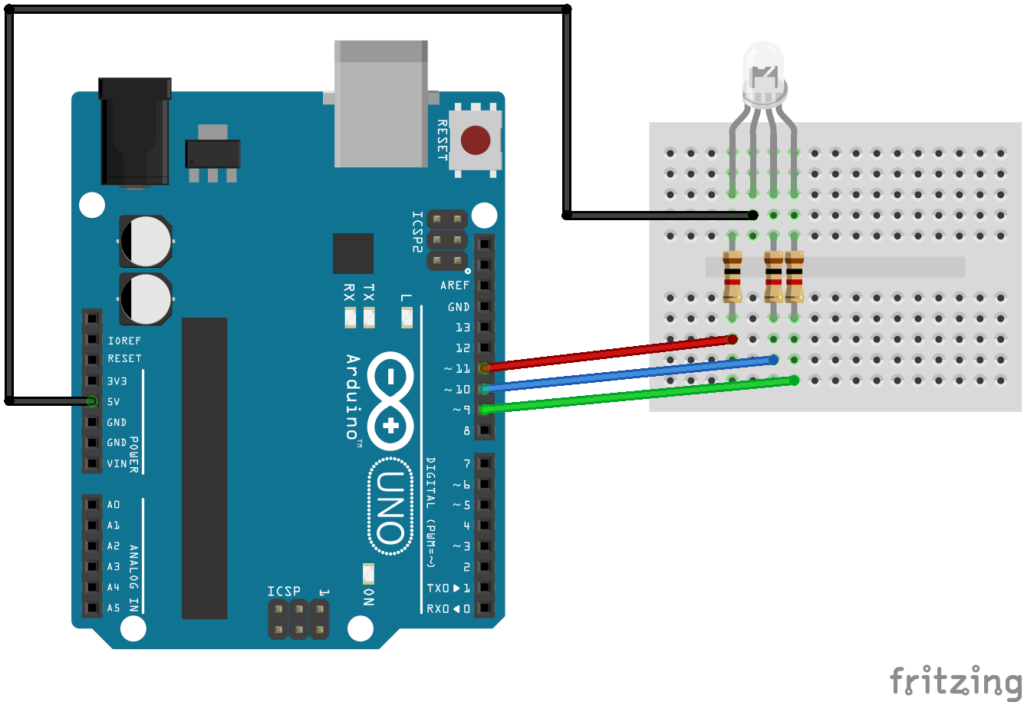# Arduino for beginners : while loop

while loops will loop continuously, and infinitely, until the expression inside the parenthesis, () becomes false. Something must change the tested variable, or the while loop will never exit. This could be in your code, such as an incremented variable, or an external condition, such as testing a sensor.
The while loop has a structure as follows:

while (test expression) {
Statement 1
Statement 2

}
test expression – a (boolean) C statement that evaluates to true or false

Lets look at some examples

Code Examples

[codesyntax lang=”cpp”]

```void setup()
{
int i = 1;

Serial.begin(9600);

while(i<=10)
{
Serial.print("i = ");
Serial.println(i);
i++;
}
}

void loop() {
}```

[/codesyntax]

Open the serial monitor and you should see the following

i = 1
i = 2
i = 3
i = 4
i = 5
i = 6
i = 7
i = 8
i = 9
i = 10

As you can see we set the variable to 1, we then test whether the variable is less than or equal to 10. If it is not then the statements will run in the brackets which is basically outputing this value via the serial monitor. Important part is that we increment the variable using i++.

This increments the value of the variable by 1, this is the same incidentally as writing i = i + 1; which is sometimes used as well. Forgetting this would cause the while loop to always run – an infinite loop. You can remove the i++ and see what happens.

Now back to the RGB led exampleNow lets fade that led again like in the for loop example

[codesyntax lang=”cpp”]

```//change the brightness of an LED
int redled = 11;
int i =0;

void setup()
{
pinMode(redled, OUTPUT); //set the LED pin as an output
Serial.begin(9600);
while(i<=255)
{
Serial.print("loop = ");
Serial.println(i);
analogWrite(redled, i);
delay(10);
i++;
}
}

void loop()
{
}```

[/codesyntax]

Lets flash that red led 10 times again

[codesyntax lang=”cpp”]

```//flash the red led of the RGB led 10 times
int redled = 11;
int i = 1; //init the variable

void setup()
{
Serial.begin(9600);
pinMode(redled, OUTPUT); //set the LED pin as an output
//this will run 10 times
while(i<=10)
{
Serial.print("loop = ");
Serial.println(i);
digitalWrite(redled, LOW); // led on
delay(500);
digitalWrite(redled, HIGH); //led off
delay(500);
i++; //dont forget this
}
}

void loop()
{
}```

[/codesyntax]

This div height required for enabling the sticky sidebar